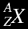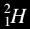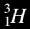# Nuclear Decay Equations

Instead of drawing the nuclei of atoms, it is easier to write about them using symbols. We write:Where X is the symbol for the element (e.g. H, Na, C, etc.)
Z is the atomic number (number of protons)
A is the mass number (number of protons + neutrons).

For example:

IsotopeSymbol Form
HydrogenDeuteriumTritiumRadioactivity MenuGo to next page